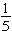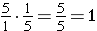# Division of Fractions and Mixed NumbersIn this lesson, you will expand your skills in fraction division. You will learn that dividing by a number and multiplying by the reciprocal of the number give the same result. Make sure you always rewrite the division problem as a multiplication problem before you try to find common factors or cancel out factors.

Look at the following problems and simplify:Both are equal to 2.

What is the relationship between 5 and?   Let’s multiply them and find out!Now do you see what the rule “Keep, Change, Flip” means?

When you divide fractions, you are multiplying by the reciprocal to get your answer.

1. Keep the first number (dividend)
2. Change division sign to multiplication
3. Flip (or invert) the second fraction

When you divide mixed numbers, follow these steps:

1. Change the mixed number to an improper fraction
2. Keep the first number
3. Change division sign to multiplication
4. Flip (multiply by the reciprocal)
5. Simplify

(source)